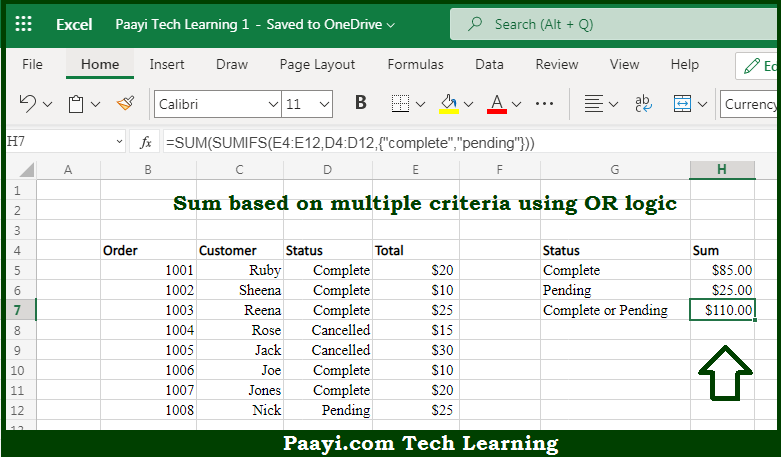# Learn How to SUMIFS With Multiple Criteria OR Logic in Microsoft Excel

Written by | 0 Comments | 638 Views

In this article, you will learn how to SUM various things in Microsoft Excel using a single/combination(s) of functions. You will also know how to SUMIFS With Multiple Criteria OR Logic and see the generic formula.

SUMIFS With Multiple Criteria OR Logic in Microsoft Excel

The main purpose of this formula is to sum based on multiple criteria using OR logic. Here we will learn how to SUMIFS with multiple criteria using the OR logic in the workbook in Microsoft Excel. That implies, with the help of a formula based on the SUMIFS and SUM function you can able to sum based on multiple criteria using OR logic. So, with the help of this formula, you can able to SUMIFS with multiple criteria using the OR logic in the workbook in Microsoft Excel.

General Formula to SUMIFS With Multiple Criteria OR Logic

=SUM(SUMIFS(sum_range,criteria_range,{"red","blue"}))

The Explanation for the SUMIFS With Multiple Criteria OR LogicSo we know that with the help of the given formula above you can be able to sum based on multiple criteria using OR logic. Here we will learn how to SUMIFS with multiple criteria using the OR logic in the workbook in Microsoft Excel. As we know that the SUMIFS function only allows AND logic that means when you provide multiple conditions, all conditions must match to be included in the result. One way can be to supply multiple criteria in an array constant. It will cause the SUMIFS function to return two results: a count for "complete" and a count for "pending", in an array result. So, with the help of this formula, you can able to SUMIFS with multiple criteria using the OR logic in the workbook in Microsoft Excel.